# Help with any of these:    21

Help with any of these:

21. Aluminium sulphate is often used by gardeners to acidify soil and can be produced by the reaction
2Al(OH)3(aq) + 3 H2SO4(aq) à Al2(SO4)3(aq)  + 6 H2O(l)
The volume of 0.275 mol/L H2SO4(aq) needed to react completely with 112 mL of 0.355 mol/l Al(OH)3(aq) is
A. 96.4 mL
B. 145 mL
C. 168 mL
D. 217 mL

22. To produce the anti cancer drug cisplatin (Pt (NH3)2Cl2(s)), a technician reacted NH3(aq) with excess K2PtCl4(aq). The reaction follows
K2PtCl4(aq) + 2 NH3(aq) à Pt(NH3)2Cl2(s) + 2 KCl(aq)
The data was recorded by the technician as follows.
Concentration of NH3(aq) used = 0.450 mol/L
Mass of filter paper = 0.725 g
Mass of filter paper and solid precipitate = 9.544 g
The volume of NH3(aq) used by the technician was
A. 32.7 mL
B. 65.3 mL
C. 131 mL
D. 141 mL

23.  When calcium reacts with nitrogen, calcium nitride is formed, as shown in the following balanced chemical equation:
3 Ca(s) + N2(g)  Ca3N2(s)
If 24.0 g of calcium and 12.0 g of nitrogen are available for this reaction, the limiting reagent is:
A.        calcium
B.        nitrogen
C.        calcium nitride
D.        both calcium and nitrogen will be completely consumed

24.  When 45 mL of 0.25 mol/L sulfuric acid is mixed with 25 mL of 0.48 mol/L calcium hydroxide, the solution
A.        is acidic
B.        is neutral
C.        is basic
D.        not enough information is given

25.  Consider the reaction equation 3 Fe(s) + 4 H2O(l)  Fe3O4(s) + 4 H2(g). If 3.0 mol Fe reacts with 1.0 mol H2O in ideal conditions, which statement is true?
A.        Fe is in excess.
B.        H2O is in excess.
C.        Both reactants are completely consumed.
D.        Not enough information is given to answer the question.
26. A group of students performed a titration analysis. The results are given in the graph below.

The indicator that the students should use to find the endpoint pH is:
A.        methyl orange
B.        methyl red
C.        bromothymol blue
D.        phenolphthalein

27.  In a titration, a 20 mL sample of NaOH(aq) was neutralized by 14.9 mL of 0.13 mol/L H2SO4(aq). The concentration of the base is
A. 8.7 mol/L
B. 0.35 mol/L
C. 0.19 mol/L
D. 0.048 mol/L

28.  For complete neutralization, 15.0 mL of 0.35 mol/L sodium hydroxide solution was required to react with 0.425 g of an acid. The possible identity of the acid is.
A. HBr (aq)
B. HCl(aq)
C. HNO3(aq)
D. H2SO4(aq)

29.  A lab group decides to test a manufacturer’s claim that an antacid product contains 200 mg of sodium bicarbonate. Each tablet was placed into an Erlenmeyer flask, 10.00 mL of water was added, and then the tablets were crushed. An indicator was added to each flask, and then each sample was titrated with 0.200 mol/L hydrochloric acid. The titration evidence was collected in the table below

Titration of 10.00 mL of NaHCO3(aq) with 0.200 mol/L HCl(aq)
Trial
1
2
3
4
11.6
21.7
31.7
41.8
0.6
11.6
21.7
31.7

Indicator colour
red
orange
orange
orange

The calculated mass of sodium bicarbonate in the antacid tablet is:
A.        169 mg
B.        173 mg
C.        84.6 mg
D.        86.5 mg

30.   During a titration, results were recorded for three trials for the complete neutralization of 0.100 mol/L potassium carbonate solution with hydrochloric acid

The Volume of HCl(aq) Required to React With a Given Volume of K2CO3(aq)

Volume (mL)
Trial 1
Trial 2
Trial 3
K2CO3(aq)
20.0
20.0
20.0
HCI(aq)
22.3
22.5
22.4

From the data, it can be calculated that the concentration of the acid is
A. 4.46 X 10–1 mol/L
B. 1.79 X 10–1 mol/L
C. 4.46 X 10–2 mol/L
D. 1.79 X 10–2 mol/L

31.  When a solution of H2S(aq) is mixed with a solution of Pb(NO3)2(aq), the ionic equation of the above reaction is
a.         Pb2+ (aq) + 2NO3–(aq)  Pb(NO3)2(aq)
b.         S2–(aq) + Pb2+ (aq)  PbS(s)
c.         2H+(aq) + S2–(aq) + Pb2+ (aq) + 2NO3–(aq)  PbS(s) + 2H+(aq) + 2NO3–(aq)
d.         S2(aq) + Pb2+ (aq) + 2NO3(aq)  PbS(s) + 2NO3(aq)

32.

In the Haber process, ammonia is formed by the reaction of nitrogen with hydrogen under high pressure and high temperature. The reaction is expressed as follows:

If 2.00 g of N2(g) reacts with excess hydrogen to produce 1.85 g of ammonia, what will the percentage yield be for the reaction?
a.         75.9%  c.         77.9%
b.         76.1%  d.         82.2%

33.  A solution of NaCl(aq) is added dropwise to a 10.0 mL sample of water from an industrial site that contains lead(II) nitrate, Pb(NO3)2(aq). A white precipitate forms immediately. What is the net ionic equation for the reaction that occurred?
A.    Na+(aq) + Cl-(aq) ® NaCl(s)
B.     Pb2+(aq) + 2NO3-(aq) + 2Na+(aq) + 2Cl-(aq) ® PbCl2(s) + 2Na+(aq) + 2Cl-(aq)
C.     Pb2+(aq) + 2NO3-(aq) + 2Na+(aq) + 2Cl-(aq) ® Pb2+(aq) + 2Cl-(aq) + 2NaCl(s)
D.    Pb2+(aq) + 2Cl-(aq) ® PbCl2(s)

NR1  When 12.71 g of copper react with 8.000 g of sulfur, 14.72 g of copper(I) sulphide is produced. What is the percentage yield in this reaction?
2Cu(s) + S(g) ® Cu2S(s)

NR2.   What mass of the reagent in excess will remain after the reaction in which
0.3604 g of H2O(l) react with 0.8000 g of O2(g)?    2H2O(l) + O2(g) ---> 2H2O2

Two suggestions to help.
1. You get help more quickly if you post just one at a time.
2. Most of the problems are either stoichiometric or limiting reagent problems. Here are examples of each; just follow the steps.
http://www.jiskha.com/science/chemistry/stoichiometry.html

http://www.jiskha.com/science/chemistry/limiting_reagent_problem.html

The titration problems can be solved by a three step process.
25 mL A + 50 mL of 0.1 B. What is molarity of A.
1. Convert B to mols. mols = M x L = ?
2. Use the coefficients in the balanced equation to convert mols B to mols A.
3. Then M A = mols A/L A.
b.

#### Earn Coin

Coins can be redeemed for fabulous gifts.

Similar Homework Help Questions
• ### thank you!! Question 23 (2 points) Give the net ionic equation (if any) for the reaction...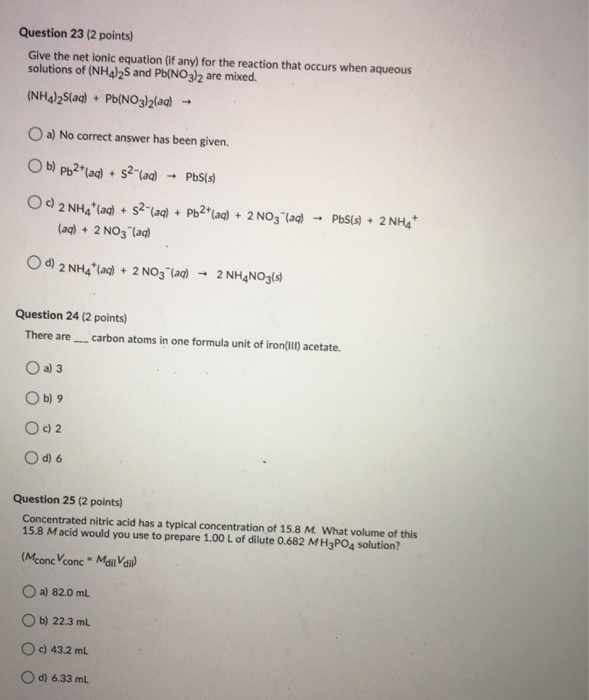thank you!! Question 23 (2 points) Give the net ionic equation (if any) for the reaction that occurs when aqueous solutions of (NH4)2S and Pb(NO3)2 are mixed. (NH4)2S(aq) + Pb(NO3)2(aq) - a) No correct answer has been given. Ob) Pb2+(aq) + 52 (aq) - PbS(s) O )2 NHA'laq) + 52" (aq) + Pb2+ (aq) + 2NO3(aq) (aq) + 2NO3(aq) Pb(s) + 2NH4 d) 2 NH4+ (aq) + 2NO3(aq) 2 NH4NO3(s) Question 24 (2 points) There are carbon atoms in one...

• ### QUESTION 1 In the commercial manufacture of nitric acid, how many moles of NO2(g) are required...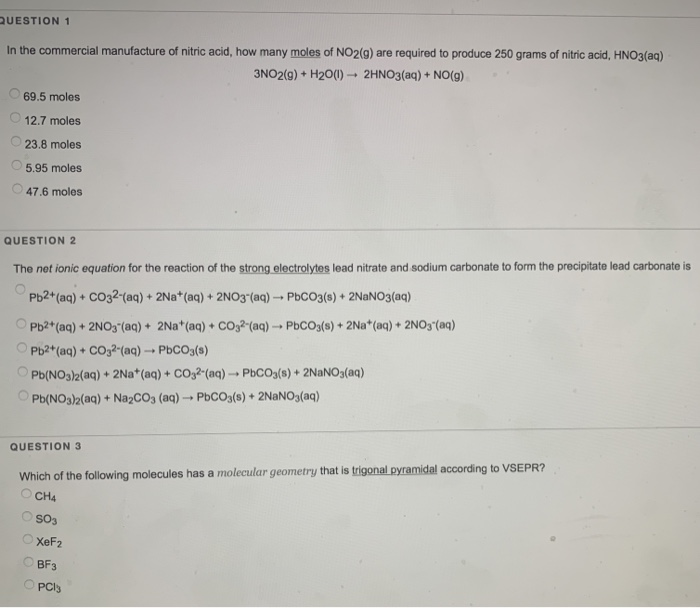QUESTION 1 In the commercial manufacture of nitric acid, how many moles of NO2(g) are required to produce 250 grams of nitric acid, HNO3(aq) 3NO2(g) + H20(1) - 2HNO3(aq) + NO(g) 69.5 moles 12.7 moles 23.8 moles 5.95 moles 47.6 moles QUESTION 2 The net ionic equation for the reaction of the strong electrolytes lead nitrate and sodium carbonate to form the precipitate lead carbonate is Pb2+(aq) + CO32-(aq) + 2Na+(aq) + 2NO3- (aq) - PCO3(s) + 2NaNO3(aq) Pb2+(aq) 2NO3"(aq)...

• ### Specific heat = 4.18 J/g*C MgO(s) + 2HCl(aq) → 2Cl(aq) + Mg(aq) + H2O(l): AH = A student added 0.250 g of Mg...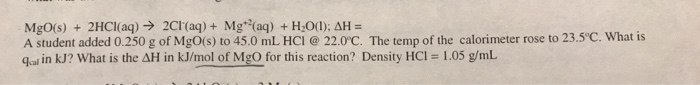Specific heat = 4.18 J/g*C MgO(s) + 2HCl(aq) → 2Cl(aq) + Mg(aq) + H2O(l): AH = A student added 0.250 g of MgO(s) to 45.0 mL [email protected] 22.0'C. The temp of the calorimeter rose to 23.5°C. What is qarin kJ? What is the AH in kJ/mol of Mgo for this reaction? Density HCI = 1.05 g/mL

• ### DETERMINATION OF A CHEMICAL FORMUL 4. If some of the calcium metal remains unreacted when you...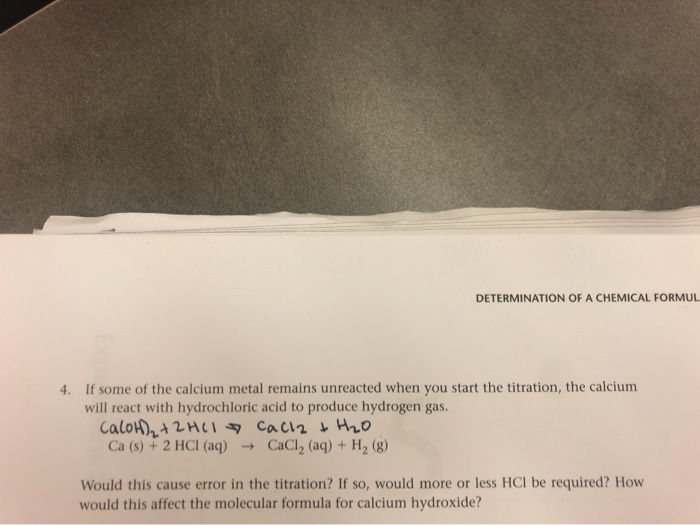DETERMINATION OF A CHEMICAL FORMUL 4. If some of the calcium metal remains unreacted when you start the titration, the calcium will react with hydrochloric acid to produce hydrogen gas. CaloH)₂ + 2HCl cacla & H2O Ca (s) + 2 HCl(aq) +CaCl2 (aq) + H2 (8) Would this cause error in the titration? If so, would more or less HCl be required? How would this affect the molecular formula for calcium hydroxide? bicarbonate that must be used to neutralize the...

• ### Please answer the questions clearly, showing all working. 1(i). Balance the following equations, and then write...

Please answer the questions clearly, showing all working. 1(i). Balance the following equations, and then write the net ionic equation (a) (NH4)2CO3(aq) + Cu(NO3)2(aq) → CuCO3(s) + NH4NO3(aq) (b) Mg(OH)2(s) + HCl(aq) → MgCl2(aq) + H2O(l) (c) BaCO3(s) + HBr(aq) → BaBr2(aq) + H2O(l) + CO2(g) 1(ii). For each reaction equation, identify the driving force of the reaction 2. What is an electrolyte? How can you experimentally differentiate between a weak electrolyte and a strong electrolyte? Name and give the...

• ### What volume of CO2(g) at 25°C and 760 Torr is produced when 1.00 kg of calcium...

What volume of CO2(g) at 25°C and 760 Torr is produced when 1.00 kg of calcium carbonate is used to neutralize a sulfuric acid spill? The equation for the reaction is CaCO3(s) + H2SO4(aq) ® CaSO4(s) + CO2(g) + H2O(l) A. 321 L B. 122 L C. 488 L D. 22.4 L E. 244 L Show steps please.

• ### Consider this equation, describing a titration you performed during the electrolytes lab in Chem 151: 2...

Consider this equation, describing a titration you performed during the electrolytes lab in Chem 151: 2 H3PO4 (aq) + 3 Ca(OH)2 (aq) → Ca3(PO4)2 (s) + 6 H2O (l) a) Calculate the volume of 0.0500 M phosphoric acid that would be required to exactly react with 20.0 mL of 0.100 M calcium hydroxide b) What mass of calcium phosphate will precipitate?

• ### 1. Lead(II) sulfide dissolves in excess nitric acid according to the equation below. Calculate the volume...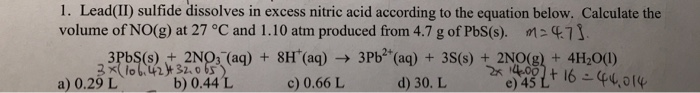1. Lead(II) sulfide dissolves in excess nitric acid according to the equation below. Calculate the volume of NO(g) at 27 °C and 1.10 atm produced from 4.7 g of PbS(s). M24.71 3Pb (s) + 2NO3(aq) + 8H(aq) 3Pb2+ (aq) + 3S(s) + 2NO(g) + 4H2O(1) 3x106.42432.065) a) 0.29 L b) 0.44 L c) 0.66 L d) 30. L 2x 4,057 + 16 - 44,014

• ### How many liters of oxygen at 23° C and 1.12 atm will be required for the...

How many liters of oxygen at 23° C and 1.12 atm will be required for the combustion of 10.0 g of methane, CH4, according to the chemical reaction below:                                  CH4 (g) + 2 O2 (g)­ ---> CO2 (g) + 2 H2O (g)   When magnesium burns in air, the reaction is described by:  2 Mg (s) + O2 (g) ---> 2 MgO (s) What mass of magnesium will react with 10.8 L of oxygen if the temperature and pressure of the...

• ### 1. The heat capacity of a calorimeter is given as 1264 J/oC.   Suppose the enthalpy of...

1. The heat capacity of a calorimeter is given as 1264 J/oC.   Suppose the enthalpy of solution for calcium chloride (MM=111 g/mol) in water is given by the thermochemical equation: CaCl2(s) + H2O(l) → Ca2+(aq) + 2Cl-(aq)   ΔH = -81.0 kJ/mol. Calculate the temperature change if 11.1 g of calcium chloride is dissolved in water in the calorimeter. 2. Given the following data, calculate ΔH for the reaction of two moles of carbon monoxide with oxygen gasto give carbon dioxide....